# Physics of a Hydrofoil

Watching a foiler for first time from far away may does not reveal, that the foiler is actually not surfing on a board that is gliding on the water. No, actually the foiler is flying above the water surface, standing on a board connected via a mast to a foil below the water surface. But how is the buoyancy – or more precise – lifting force of a foil determined? How is the physics of a hydrofoil? Let us have a quick look at it and try to explain it in a simplified approach. The engineers and physicist among you hopefully accept that we simplified the approach.

Basically, it does not matter if you look at a wing of an aircraft or at a wing of foilboard that is moving in the water, also called hydrofoil. However, the medium in that the wing is acting has different physical properties, e.g. the density of water is different than the density of air, but the physical behavior is similar.

On a hydrofoil are basically acting four different forces:

• The weight force
• Lift force
• Propulsion (thrust force)
• Drag force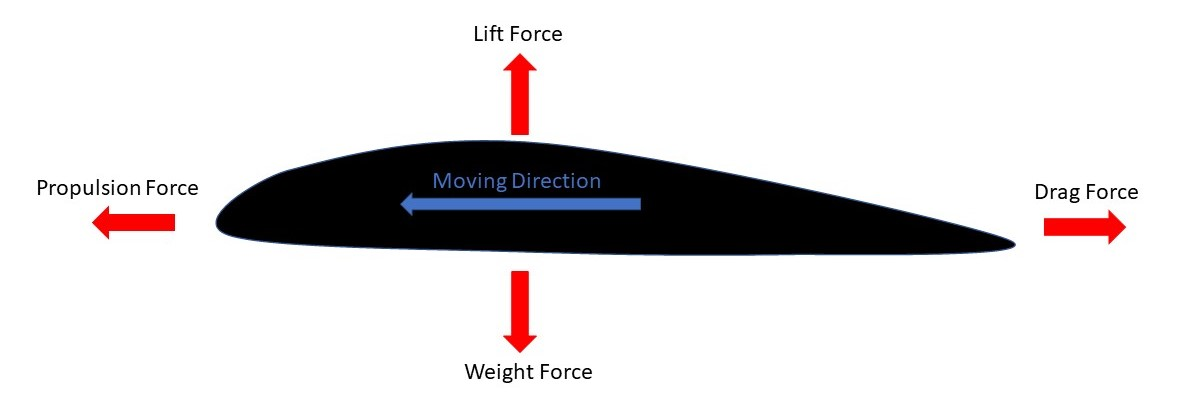The lift force is the force that is lifting up the ‘system’, respectively is counteracting the weight force, which consists of the weight of the rider as well as the weight of the foiling setup (board, mast, fuselage, front wing, back wing). The drag force is the drag acting on the foil when moving it through the water. The drag force is acting against (counteracting) the thrust force. Let us now have a look how the different forces are calculated.

The force induced by the rider and the weight of the equipment, let us call it the weight force, is calcuated by: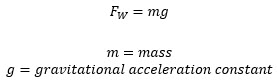The lift force is dependent on the densitiy of the water, the speed of the foil relative to the water, the area of the wing as well as the lift coefficient of the wing.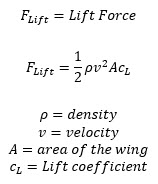The drag force is calculated similar to the lift force and is dependent on the densitiy of the water, the speed of the foil relative to the water, the area of the wing as well as the drag coefficient of the wing.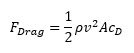The propulsion force will be calculated different on how the propulsion is induced. For our case, let us keep it at the fact the propulsion force must be equal to the drag force to keep the system at a constant speed.

Now we have the formulas together do some calcuations. As an example, let us calculate the lift force of a pumpfoil setup I frequently use.

I like a lot the Indiana 1100P Foil Front Wing in combination with the Indiana Stabilizer 420. This foil generates a lot of lift at low speeds and is ideal to start with pumpfoiling.

Let us assume that we are pumping in fresh water at a speed of ~10 km/h:

• The wing area of the Indiana 1100P Front Wing is 2300 square cm
• The density of fresh water is approximately 997 kg per cubic meter (dependent on the temperature)
• The lifting coefficient is not known, however comparing with other foils with known lifting coefficient and assumption of a certain angle of attack, let us assume it is 1.1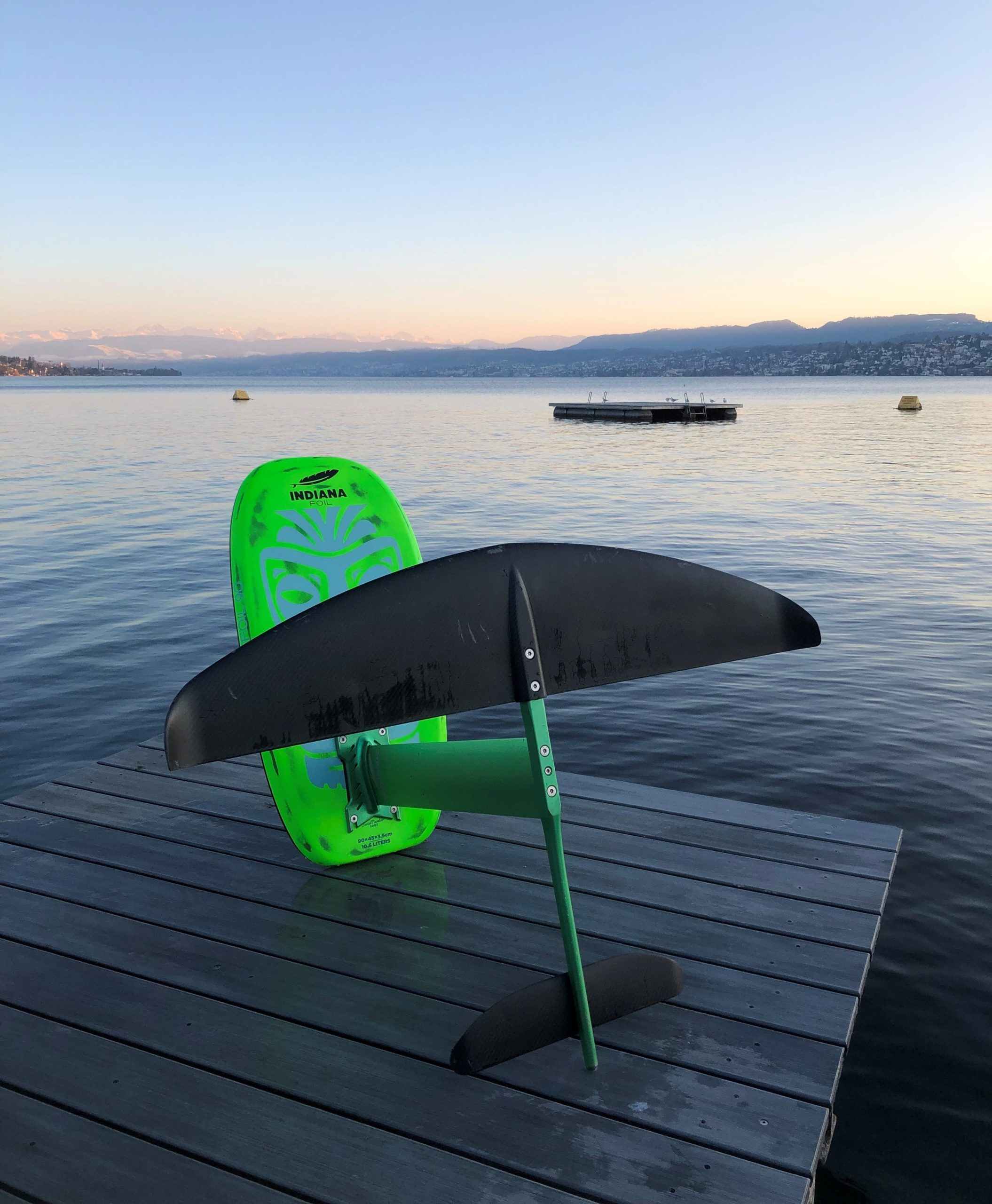Now we can apply the equation for the lift force: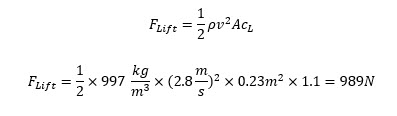As we can see, the lift force generated is ~989 Newton which will be enough to lift a rider 0f around 85-90 kg together with the foil setup. Please note that we have only calculated the lift generated by the front wing. We have not considered any impact of the back wing also not discussed the propulsion and drag force. However, it provides a nice first estimation about the lift generated by a decent pumpfoiling setup.

So most of you now will ask: Hey but what happens when you change the pitch of the wing/foil?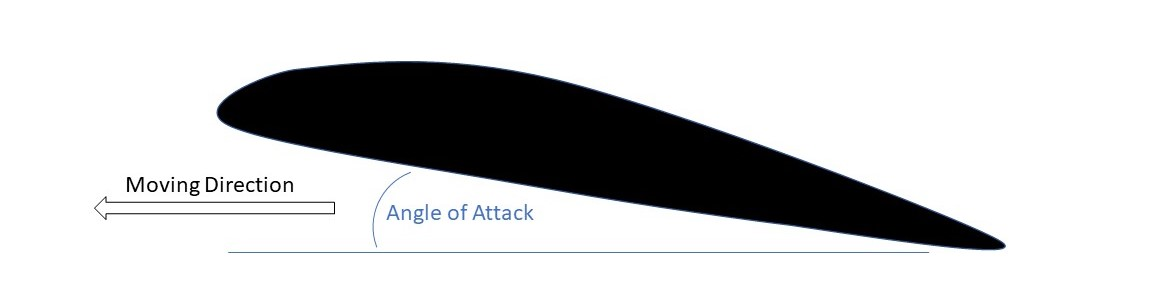Well, the pitch or angle of attack hast an impact on the lifting force as it changes the pressure difference between the upper and lower side of the foil. In the equation we used to calculate the lift force, the angle of attack is included in the lift coefficient. There is a non-linear correlation between the angle of attack and lift force coefficient. Thereby, the angle of attack is measured as the angle angle between the moving direction (opposite to the water or air flow) and the alignment of the foil.

In case you increase the angle of attack, the lift coefficient will increase, however only to a certain level. In case the angle becomes too big, it will imply a stall.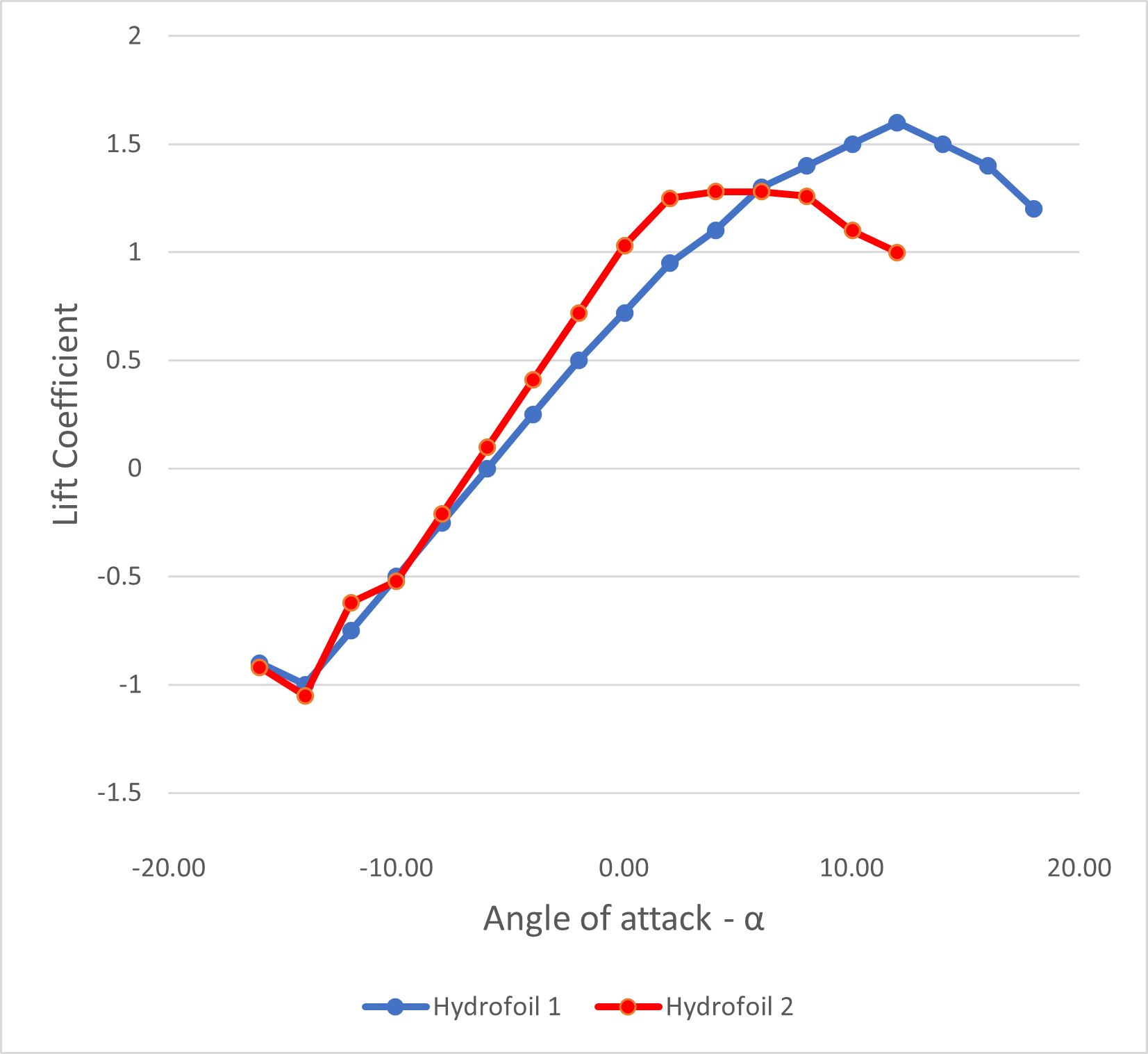In case you are interested more into physics of a hydrofil, you can find quite some information in the internet. Just ask Google for help.

More specific information about different foil shapes and related specifications, you can find also find in the internet, e.g. on Airfoil Tools .